Learning Library

12 Times Table Worksheets - One through Twelve

Hoping to help your kid master those tricky times tables? This straightforward series is the perfect way to get in some much-needed multiplication practice.

Click on a worksheet in the set below to see more info or download the PDF.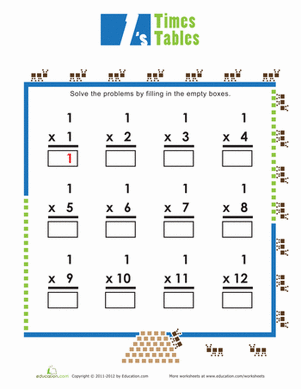Times Tables: Ones

The ants go marching one by one, hurrah! Get some practice multiplying by one with this times tables practice sheet.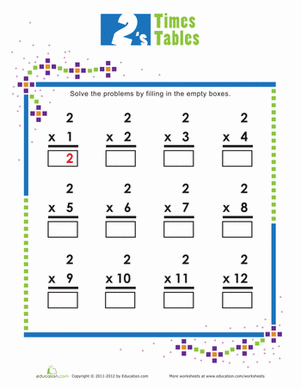Times Tables: Twos

How well does your child know her times tables? Get some practice multiplying by two with this times tables practice sheet.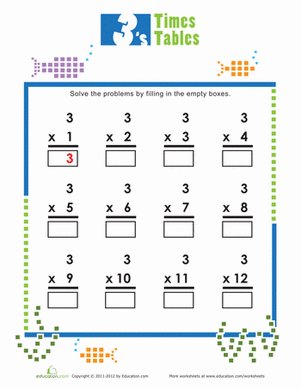Times Tables: Threes

Give your student some practice multiplying by threes with this times tables practice sheet.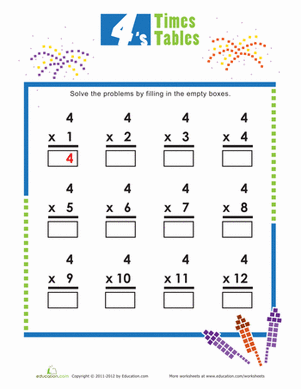Times Tables: Fours

How well does your child know her times tables? Get some practice multiplying by fours with this times tables practice sheet.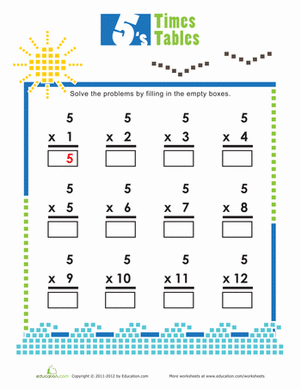Times Tables: Fives

See how well your child knows her times tables, and give her some practice multiplying by fives with this times tables practice sheet.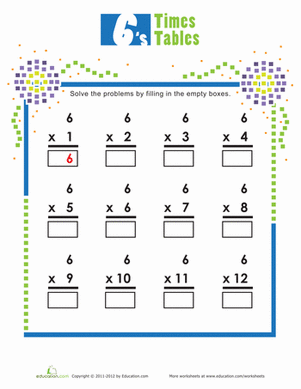Times Tables: Sixes

How well does your child know her times tables? Get some practice multiplying by sixes with this times tables practice sheet.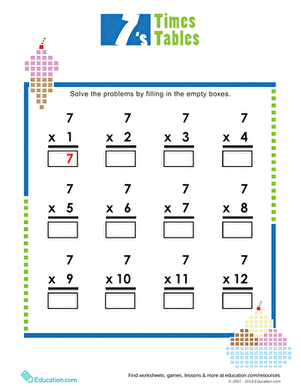Times Tables: Sevens

Test your child on her times tables knowledge, and practice multiplying by sevens with this times tables practice sheet.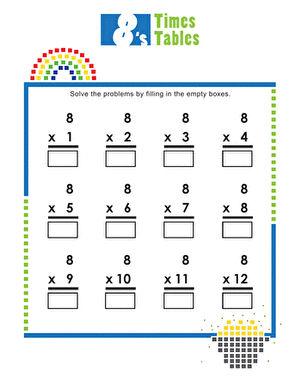Times Tables: Eights

Eight is great, but a little tricky to multiply! Give your student some practice multiplying by eights with this times tables practice sheet.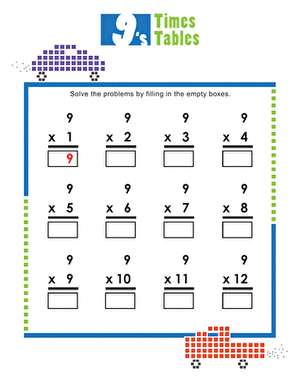Times Tables: Nines

Nine is fine, especially when multiplied! Give your student some practice multiplying by nine with this times table worksheet.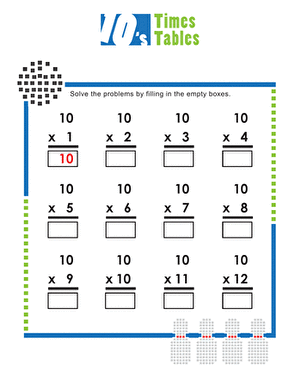Times Tables: Tens

Whew, time for the easy tens! Get some practice multiplying by tens with this times tables practice sheet.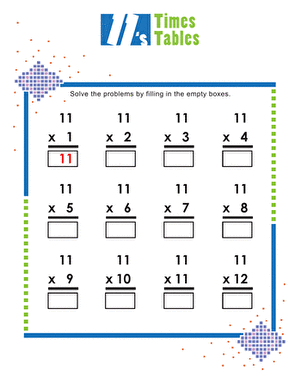Times Tables: Elevens

Okay, okay, eleven is definitely heaven, more so than seven! Have your student brush up on her times tables with this fun practice sheet.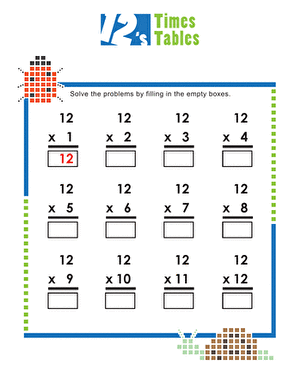Times Tables: Twelves

Help your student work on those tricky twelves times tables with this practice sheet.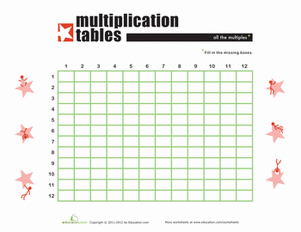Blank Times Table Grid

For students studying times tables, this blank times table sheet may be the best possible tool to help you memorize!

Want to download this whole set as a single PDF?

Create new collection

0

New Collection>

0 items

What could we do to improve Education.com?

Please note: Use the Contact Us link at the bottom of our website for account-specific questions or issues.

What would make you love Education.com?